Processing ......FreeComputerBooks.com Links to Free Computer, Mathematics, Technical Books all over the World

Applied Mathematical Programming
Top Free JavaScript Books 🌠 - 100% Free or Open Source!
• Title: Applied Mathematical Programming
• Author(s) Man-Keun Kim, Bruce A. McCarl, Thomas H. Spreen
• Publisher: Utah State University, Texas A&M University, and University of Florida
• Hardcover: N/A
• eBook: PDF
• Language: English
• ISBN-10: N/A
• ISBN-13: N/A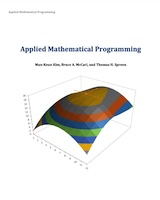Book Description

This book emphasize model-formulation and model-building skills as well as interpretation of computer software output. Focusing on deterministic models, this book is designed for the operations research sequence. It has a strong computer orientation and emphasizes model-formulation and model-building skills.

• N/A
Reviews, Ratings, and Recommendations: Related Book Categories: Read and Download Links:Similar Books:
•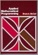Applied Mathematical Programming Using Algebraic Systems

This book is both a reference guide and a text for a course on Applied Mathematical Programming. The material presented will concentrate upon conceptual issues, problem formulation, computerized problem solution, and results interpretation.

•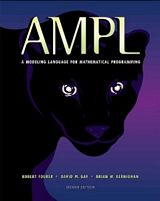AMPL: A Modeling Language for Mathematical Programming

This book is a complete guide to AMPL for modelers at all levels of experience. It begins with a tutorial on widely used linear programming models, and presents all of AMPL's features for linear programming with extensive examples.

•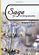Sage for Undergraduates (Gregory V. Bard)

This book is a gentle introduction to SageMath for undergraduate students toward the end of Calculus or higher-level course work such as Multivariate Calculus, Differential Equations, Linear Algebra, or Math Modeling.

•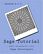Sage Tutorial (William Stein)

This tutorial is the best way to become familiar with SageMath in only a few hours. It explains how to use the free and open source mathematical software package Sage. Both binaries and source code are available for SageMath from the download page.

•Mathematica® Programming: an Advanced Introduction

Starting from first principles, this book covers all of the foundational material needed to develop a clear understanding of the Mathematica® language, with a practical emphasis on solving problems.

•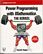Power Programming with Mathematica® (David B. Wagner)

This book unpacks Mathematica® for programmers, building insights into programming style via real world syntax, real world examples, and extensive parallels to other languages.

•Solving PDEs in Python: The FEniCS Tutorial I (H. Langtangen)

This book offers a concise and gentle introduction to finite element programming in Python based on the popular FEniCS software library. Using a series of examples, it guides readers through the essential steps to quickly solving a PDE in FEniCS.

•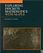Exploring Discrete Mathematics With Maple (Kenneth H. Rosen)

This is is a supplement in discrete mathematics to concentrate on the computational aspects of the computer algebra system Maple. Detailed instructions for the use of Maple are included in an introductory chapter and in each subsequent chapter.

•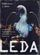LEDA: A Platform for Combinatorial and Geometric Computing

LEDA is a library of efficient data types and algorithms and a platform for combinatorial and geometric computing. This book, written by the main authors of LEDA, is the definitive account of how the system operates and how it can be used.

•Certified Programming with Dependent Types on Sat Nov 12, 2016

This book provides an introduction to the Coq software for writing and checking mathematical proofs. It takes a practical engineering focus throughout, emphasizing techniques that will help users to build, understand, and maintain large Coq code changes.

•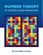Number Theory: In Context and Interactive, using SageMath

This is a textbook for an upper-level number theory course, with a clear vision to expose students to the connections to all areas of mathematics, and nearly every concept can be visualized or experimented with using the mathematics software SageMath.

•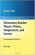Elementary Number Theory using SageMath (William Stein)

This is a book about prime numbers, congruences, secret messages, and elliptic curves that you can read cover to cover. Many numerical examples are given throughout the book using the SageMath mathematical software.

•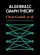Explorations in Algebraic Graph Theory with SageMath

This book aims to express properties of graphs in algebraic terms, then to deduce theorems about them. It tackles the applications of linear algebra and matrix theory to the study of graphs; algebraic constructions such as adjacency matrix, using the SageMath.

•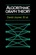Algorithmic Graph Theory using SageMath (David Joyner, et al)

This is an introductory book on algorithmic graph theory. Theory and algorithms are illustrated using the Sage open source mathematics software. It's especially suitable for computer scientists and mathematicians interested in computational complexity.

Book Categories
 :All CategoriesTop Free BooksRecent BooksMiscellaneous BooksComputer EngineeringComputer LanguagesComputer ScienceData Science/DatabasesJava and Java EE (J2EE)Linux and UnixMathematicsMicrosoft and .NETMobile ComputingNetworking and CommunicationsSoftware EngineeringSpecial TopicsWeb Programming
Other Categories